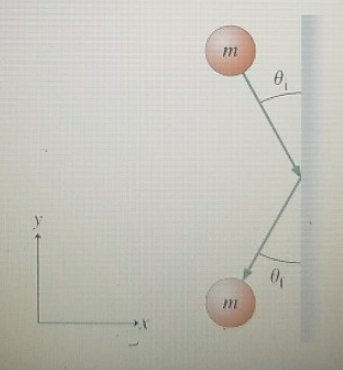# Problem:  A ball of mass m moving with velocity v, strikes a vertical wall as shown in (Figure 1). The angle between the ball's initial velocity vector and the wall is θ1 as shown on the diagram, which depicts the situation as seen from above. The duration of the collision between the ball and the wall is Δt, and this collision is completely elastic. Friction is negligible, so the ball does not start spinning. In this idealized collision, the force exerted on the ball by the wall is parallel to the x axis.What is the final angle (θf) that the ball's velocity vector makes with the negative y axis?

###### FREE Expert Solution

We'll use the law of conservation of momentum, which states that the total momentum of an isolated system remains constant.

pi = pf

Initial momentum:

pi = mvisinθi - mvicosθi

Final momentum:

pf = - mvfsinθf - mvfcosθf

Since the Collison is perfectly elastic, the initial velocity is equal to the final velocity.

95% (472 ratings)###### Problem Details

A ball of mass m moving with velocity v, strikes a vertical wall as shown in (Figure 1). The angle between the ball's initial velocity vector and the wall is θ1 as shown on the diagram, which depicts the situation as seen from above. The duration of the collision between the ball and the wall is Δt, and this collision is completely elastic. Friction is negligible, so the ball does not start spinning. In this idealized collision, the force exerted on the ball by the wall is parallel to the x axis.What is the final angle (θf) that the ball's velocity vector makes with the negative y axis?